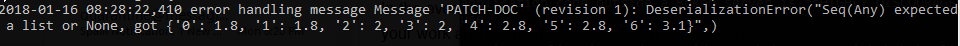/ bokeh Public

# DeserializationError when editing Data Table#7417

Closed
opened this issue Jan 16, 2018 · 7 comments · Fixed by #8310
Closed

# DeserializationError when editing Data Table #7417

opened this issue Jan 16, 2018 · 7 comments · Fixed by #8310
Labels
Milestone

### pdmich commented Jan 16, 2018

 Current environment: Bokeh 0.12.14dev3 (although I think the same error occurs in 0.12.13) Python 3.6 Windows 10 Google Chrome When editing or clicking on cells in a data table bokeh will display a `DeserializationError`:This can be tested with the data_table.py example on the bokeh github. Open that server up and then click on a cell and the error should appear. Not sure where this is coming from, but I do believe it happened somewhere after 0.12.10 (which doesn't produce the error). The text was updated successfully, but these errors were encountered:

### mattpap commented Jan 16, 2018

 Looks like a typed array.

### bryevdv commented Jan 30, 2018

 Binary array transport landed in `0.12.9` so it's not directly that.

### bryevdv commented Jan 30, 2018

 Also, that example is gone (all the bad examples of `bokeh.client` "app outside the server" were removed) but here is an updated `export_csv` that shows the issue: ``````import pandas as pd from bokeh.layouts import row, widgetbox from bokeh.models.widgets import Select, MultiSelect from bokeh.plotting import curdoc, figure from bokeh.models import ColumnDataSource, LabelSet from bokeh.models.ranges import FactorRange from bokeh.io import show df = pd.DataFrame(data={ 'category': ['e', 'e', 'f', 'f'], 'subcategory': ['a', 'b', 'c', 'd'], 'number': [1, 2, 3, 4], 'number2': [5, 6, 7, 8] }) df['cat_sub'] = list(zip(df.category, df.subcategory)) source = ColumnDataSource(df) p = figure(plot_width=800, y_range=FactorRange(factors=list(df.cat_sub)), title='sweet', plot_height=500) h1 = p.hbar(right="number", y="cat_sub", color='darkgrey', height=0.8, source=source) labels = LabelSet( text='number', text_font_size='9pt', level='glyph', x='number', y='cat_sub', x_offset=3, y_offset=-6.5, source=source) p.add_layout(labels) def update_source(): df2 = df.copy() df3 = df2[ df2.category.isin(cats.value) & df2.subcategory.isin(subcats.value)] print(df3) df4 = df3.reindex(columns=['category', 'subcategory', nums.value]) print(df4) df4['cat_sub'] = list(zip(df4.category, df4.subcategory)) source.data = source.from_df(df4) print(source.data) def update_glyph(): h1.glyph.right = nums.value labels.text = nums.value labels.x = nums.value def update(attr, old, new): update_source() update_glyph() r.children = update_glyph() catlist_u = df['category'].unique().tolist() subcatlist_u = df['subcategory'].unique().tolist() cats = MultiSelect(title='Category', value=['f'], options=catlist_u) subcats = MultiSelect(title='Subcategory', value=['a'], options=subcatlist_u) nums = Select(title='Value', value='number2', options=['number', 'number2']) cats.on_change('value', update) subcats.on_change('value', update) nums.on_change('value', update) w = widgetbox([cats, subcats, nums]) r = row([w, p], sizing_mode="scale_width") curdoc().add_root(r) curdoc().title = "test" ``````

### mattpap commented Jan 30, 2018 • edited

 I was able to reproduce this with some app examples. I will try to fix it tomorrow.

### bryevdv commented Jan 30, 2018 • edited

 FYI a potential workaround for now at least is to use python lists for a datatable source: ``````def update(): current = df[(df['salary'] >= slider.value) & (df['salary'] <= slider.value)].dropna() source.data = { 'name' : list(current.name), 'salary' : list(current.salary), 'years_experience' : list(current.years_experience), } ``````

### smilerz commented Mar 7, 2018

 Note to anyone that uses the workaround @bryevdv suggested: You have to either drop or fill or otherwise change any NaN or NaT values in your DataFrame before assigning them to the list. Otherwise the workaround seemed to correct the issue I was having.

### Karel-van-de-Plassche commented Jun 17, 2018

 Just to let you know this is still there in `0.13.0dev11'. A minimal(er) example: ```import numpy as np from bokeh.models import ColumnDataSource from bokeh.io import curdoc, output_notebook, push_notebook, show from bokeh.models.widgets import DataTable, TableColumn, StringFormatter data = dict( X = np.linspace(0, 10), # Wrap with list() to remove the unhandled error Y = np.linspace(10, 20) # Wrap with list() to remove the unhandled error ) source = ColumnDataSource(data) columns = [ TableColumn(field="X", title="X",formatter=StringFormatter(font_style="bold",text_color="midnightblue")),TableColumn(field="Y", title="Y",formatter=StringFormatter(font_style="bold",text_color="midnightblue")), ] data_table = DataTable(source=source, columns=columns, fit_columns=True, width=850, height=280, editable=True) curdoc().add_root(data_table)```